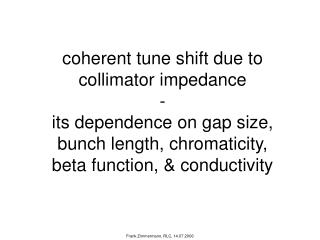DownloadDownload PresentationCu

# Cu

Télécharger la présentation## Cu

- - - - - - - - - - - - - - - - - - - - - - - - - - - E N D - - - - - - - - - - - - - - - - - - - - - - - - - - -
##### Presentation Transcript

1. coherent tune shift due to collimator impedance - its dependence on gap size, bunch length, chromaticity,beta function, & conductivity Frank Zimmermann, RLC, 14.07.2006

2. sC=105W-1m-1 impedance of single flat collimator sCu=5.9x107W-1m-1 b=70 m b=6 s s=192 mm d=3 cm 7 TeV B-L theory C Cu C Cu Frank Zimmermann, RLC, 14.07.2006

3. variation of impedance for 2 times smaller gap carbon factor 23 factor 22 factor 21 factor 23 factor 22 Frank Zimmermann, RLC, 14.07.2006

4. Gaussian weight functions Q’=0 Q’=10 m=0 Q’=10 m=1 Frank Zimmermann, RLC, 14.07.2006

5. coherent coupled-bunch head-tail tune shifts Frank Zimmermann, RLC, 14.07.2006

6. coherent tune shift – b=70 m, b=6s modulus vs. mode number m=0, Q’=0 m=0, Q’=10 m=1, Q’=10 ImQ vs mode # ReQ vs mode # Frank Zimmermann, RLC, 14.07.2006

7. coherent tune shift – b=70 m, b=6s m=1, Q’=10, all modes unstable m=0, Q’=0, half of the modes unstable m=0, Q’=10, all modes damped Frank Zimmermann, RLC, 14.07.2006

8. modes with largest DQ or ImDQ always 0 or 3564 changes by up to ~250 modes Frank Zimmermann, RLC, 14.07.2006

9. maximum tune shift |DQ| vs. gap, m=0, Q’=0, b=70 m variation with conductivity Q’=0, m=0 computed tune shifts carbon fitted curves copper ~1/b2.7~1/b3 fitted curves ~1/b2.3~1/b2 Frank Zimmermann, RLC, 14.07.2006

10. maximum growth rate ImDQ vs. gap for m=0, Q’=0 carbon computed growth rates fit result fitted curve where b is the half gap size nearly inversely linear dependence on gap size! Frank Zimmermann, RLC, 14.07.2006

11. maximum tune shift |DQ| vs. gap for m=0, Q’=0 & 10 variation with chromaticity carbon m=0 computed tune shifts Q’=0 fitted curves Q’=10 almost ~1/b3! fitted curves Frank Zimmermann, RLC, 14.07.2006

12. maximum tune shift |DQ| vs. gap for m=0 & 1, Q’=5 variation with head-tail mode carbon Q’=5 computed tune shifts m=0 fitted curves m=1 almost ~1/b3! fitted curves Frank Zimmermann, RLC, 14.07.2006

13. maximum tune shift |DQ| vs. gap, m=0, Q’=0, b varying variation with beta function carbon Q’=0, m=0 computed tune shifts b=70m fitted curves b=700m almost ~1/b3! fitted curves Frank Zimmermann, RLC, 14.07.2006

14. tune shift decrease for 10x larger b ~ about factor2 carbon, Q’=0, m=0 Frank Zimmermann, RLC, 14.07.2006

15. maximum tune shift |DQ| vs. gap, m=0, Q’=0, b=70 m variation with bunch length Q’=0, m=0 carbon computed tune shifts fitted curves sz=3.77 cm sz=7.55 cm fitted curves ~1/b3! Frank Zimmermann, RLC, 14.07.2006

16. maximum tune shift |DQ| vs. gap, m=0, Q’=0, b=70 m variation with bunch length, #bunches, conductivity, bunch charge Q’=0, m=0 computed tune shifts carbon, 2808 bunches, sz=7.55 cm, Nb=1.15x1011 fitted curves copper, 5616 bunches, sz=3.77 cm, Nb=1.7x1011 ~1/b2.7 fitted curves ~1/b2.2 Frank Zimmermann, RLC, 14.07.2006

17. correction factor from nonlinear wake components derived from A. Piwinski’s wake field, in “Impedance of Elliptical Vacuum Chambers,” DESY 94-068, Eq. (52) Frank Zimmermann, RLC, 14.07.2006

18. nonlinear correction vs. gap NLC round beam b/s Frank Zimmermann, RLC, 14.07.2006

19. conclusions • for carbon jaw DQ ~1/b2.75 , for Cu jaw ~1/b2.5 • value for Cu almost 10 times smaller • weak dependence on b: DQ ~1/b0.25 • halving bunch length increases DQ by ~50% • LHC upgrade with half sz, 1.7x1011 ppb, 5616 bunches, and Cu collimators → 1/3 tune shift of nominal LHC with C jaws • correction from nonlinear wake field a few percent for half gaps of 6s or larger Frank Zimmermann, RLC, 14.07.2006